Notebook

When short-term interest rates increase is the overall stock market (SPY) more volatile? -> When short-term rates increase does SPY change more than normal?

We will answer the question by breaking up our data into two populations, one of stock market returns when interest rates decrease or stay the same, the other of stock market returns when interest rates increase. Our null and alternative hypotheses will be based on the variances of these populations.

#### Alternative Hypothesis: If short-term interest rates increase, then stock market returns will be more volatile.Â¶

Reworded, the null and alternative hypotheses are this:

#### Alternative Hypothesis: The variance of market returns when interest rates rise is greater than the variance of market returns when interest rates decrease or stay the same.Â¶

What 'interest rates' are we referencing? What are the immediate impacts of these changes? http://www.foundationsforliving.org/articles/foundation/fedraiselower.html

How does the Federal Reserve change interest rates? https://www.thebalance.com/how-does-the-fed-raise-or-lower-interest-rates-3306127

In :
import numpy as np
import pandas as pd
import statsmodels.api as sm
import matplotlib.pyplot as plt
import scipy.stats as stats
# If the observations are in a dataframe, you can use statsmodels.formulas.api to do the regression instead
from statsmodels import regression
from statsmodels.stats.stattools import jarque_bera

In :
# Set start and end dates for data
start = '2002-06-14'
end = '2017-06-14'

# Load S&P 500, 30-day Fed Funds Futures and 5-year Treasury Note pricing data
SPY = get_pricing('SPY', fields='price', start_date=start, end_date=end)
FF = get_pricing('FF', fields='price', start_date=start, end_date=end)
FV = get_pricing('FV', fields='price', start_date=start, end_date=end)

# Import Effective Fed Funds Rate from FRED (URL at bottom of document)
data = local_csv('DFF.csv')

In :
# Because the SPY, FF, FV series only include market days, we will use the ffill to
# fill in missing values for the weekends and holidays which fedfunds already has
calendar_dates = pd.date_range(start=start, end=end, freq='D', tz='UTC')
SPY = SPY.reindex(calendar_dates, method='ffill')
FF = FF.reindex(calendar_dates, method='ffill')
FV = FV.reindex(calendar_dates, method='ffill')

# Let's match the length and dates of the different data sets
fedfunds = data["DFF"][-(len(calendar_dates)):]
fedfundsdates = data["DATE"][-(len(calendar_dates)):]
if (len(SPY) == len(FF)) and (len(FF) == len(FV)) and (len(FV) == len(fedfunds)):
print "The length of all variables is now " + str(len(SPY))
print "SPY spans from " + str(SPY.index) + " to " + str(SPY.index[-1])
print "FedFunds spans from " + str(fedfundsdates.iloc) + " to " + str(fedfundsdates.iloc[-1])

The length of all variables is now 5480
SPY spans from 2002-06-14 00:00:00+00:00 to 2017-06-14 00:00:00+00:00
FedFunds spans from 2002-06-14 to 2017-06-14


Now that the variables are all adjusted for the same days and time span, we need to adjust the values to a weekly

In :
# Get the percentage change values (multiplacative returns)
spy_returns = SPY.pct_change()[1:]
ff_returns = FF.pct_change()[1:]
fv_returns = FV.pct_change()[1:]
fedfunds_returns = fedfunds.pct_change()[1:]
fedfunds = fedfunds[1:]
fedfunds.index = spy_returns.index
fedfunds_returns.index = spy_returns.index

In :
# See what the fed funds rates look like over 15 years
plt.plot(fedfunds)
plt.legend(["Fed Funds Interest Rate"]);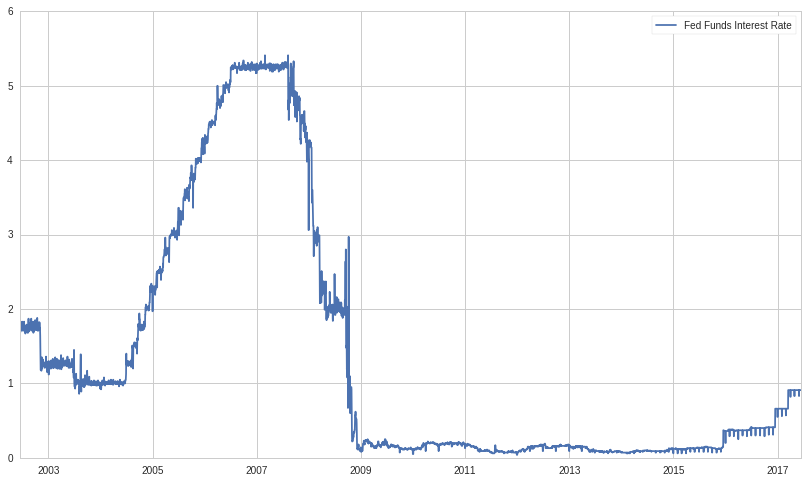In :
# See what SPY ETF looks like over the past 15 years
plt.plot(SPY)
plt.legend(["S&P 500"]);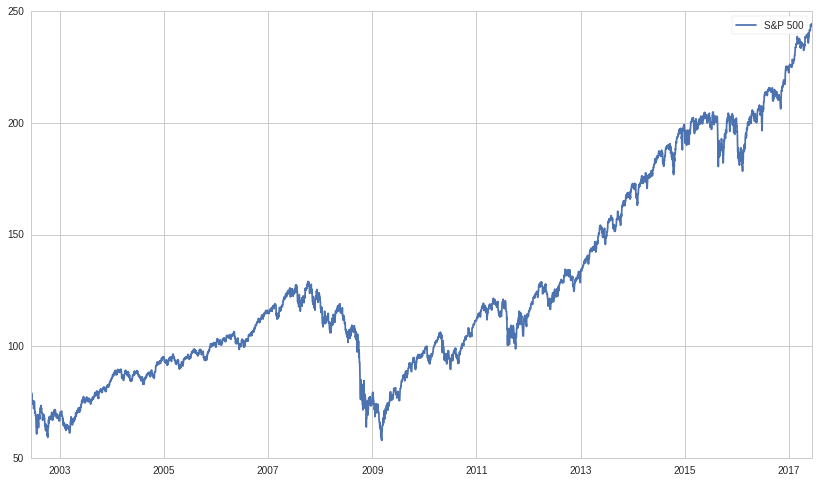In :
# Create a data frame with all the variables inside it indexed to the same timedate
df = pd.DataFrame({'SPYReturns' : spy_returns,
'SPY' : SPY,
#                   'FF' : ff_returns,
#                   'FV' : fv_returns,
'FedFundsReturns' : fedfunds_returns,
'FedFunds' : fedfunds})
#Let's ignore FF and FV until we do the rest first
# Now we can index all variables along the same datetime or value or boolean index
print df[df['FedFundsReturns']>0].tail(3)
v1 = df[df['FedFundsReturns']<=0]['SPYReturns']
v2 = df[df['FedFundsReturns']>0]['SPYReturns']

                           FedFunds  FedFundsReturns     SPY  SPYReturns
2002-06-17 00:00:00+00:00      1.82         0.040000  79.032    0.028621
2002-06-20 00:00:00+00:00      1.75         0.035503  76.765   -0.013062
2002-06-24 00:00:00+00:00      1.77         0.017241  75.617    0.005011
FedFunds  FedFundsReturns     SPY  SPYReturns
2017-04-03 00:00:00+00:00      0.91         0.109756  235.37   -0.001485
2017-05-01 00:00:00+00:00      0.91         0.096386  238.65    0.002268
2017-06-01 00:00:00+00:00      0.91         0.096386  243.33    0.007912


Graph two scatter plots of the SPYReturns v. FedFundsReturns; the first plot is of the data points with FedFunds decreasing or staying the same while the second is of those with FedFunds increasing.

Remember the null and alternative hypotheses, we are looking at increased variance in the returns, not positive or negative change.

In :
plt.scatter(v1,df[df['FedFundsReturns']<=0]['FedFundsReturns']);
plt.legend(["SPYReturns and FedFundsReturns"])
plt.title(["FedFundsReturns <= 0"]);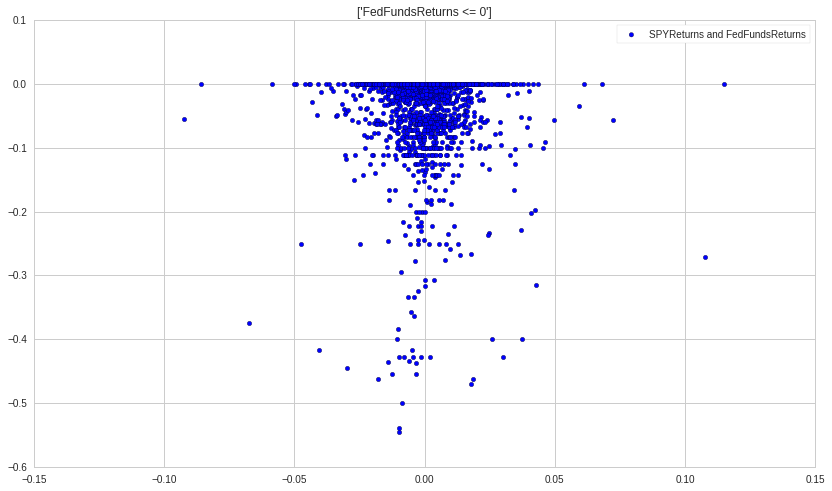In :
plt.scatter(v2,df[df['FedFundsReturns']>0]['FedFundsReturns']);
plt.legend(["SPYReturns and FedFundsReturns"])
plt.title(["FedFundsReturns > 0"]);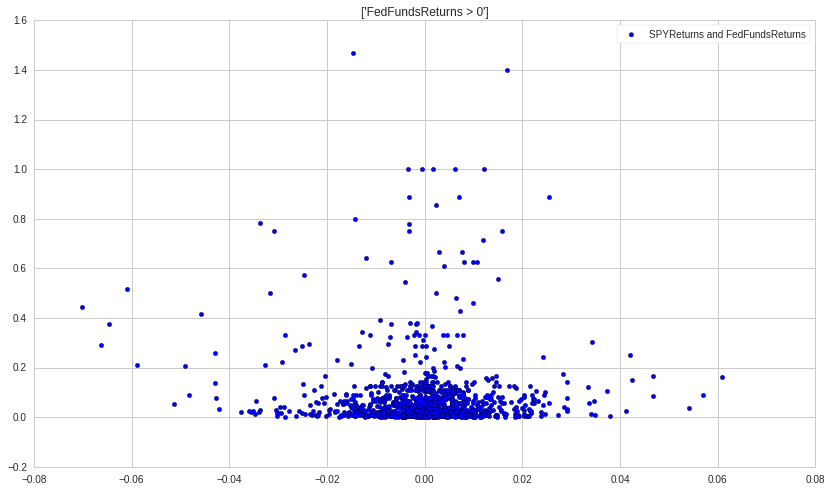The second plot appears to have a wider dispersion of points while the first one is more narrow. Let's look at the summary statistics and plot the histograms of the SPY returns in each population

In :
def summary(x):
print "Mean:   " + str(x.mean())
print "SD:     " + str(x.std())
print "Skew:   " + str(x.skew())
print "Kurt:   " + str(x.kurtosis())
print "Median: " + str(x.median())
print x.describe()[3:];


When we run the summary statistics, we want to see some differences in the stats values printed. These are just two slices from the same variable, so finding a determining factor which splits it into two different data sets is interesting.

In :
plt.hist(v1)
plt.legend(["SPYReturns"])
plt.title(["FedFundsReturns <= 0"]);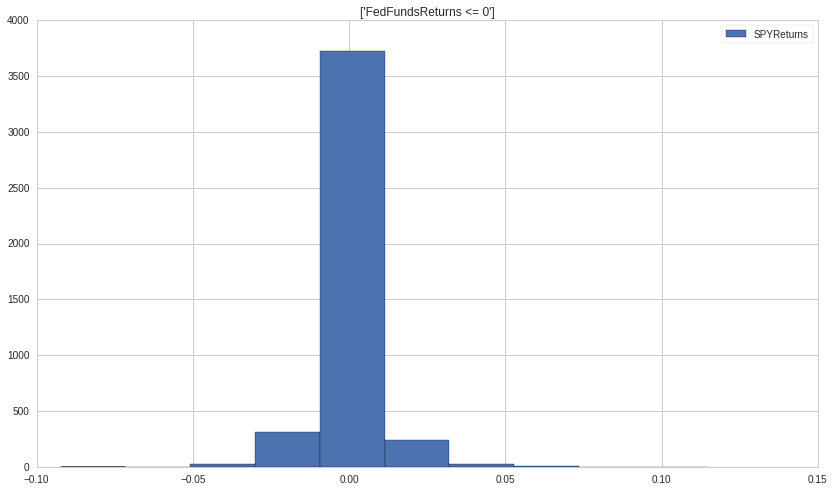In :
plt.hist(v2)
plt.legend(["SPYReturns"])
plt.title(["FedFundsReturns > 0"]);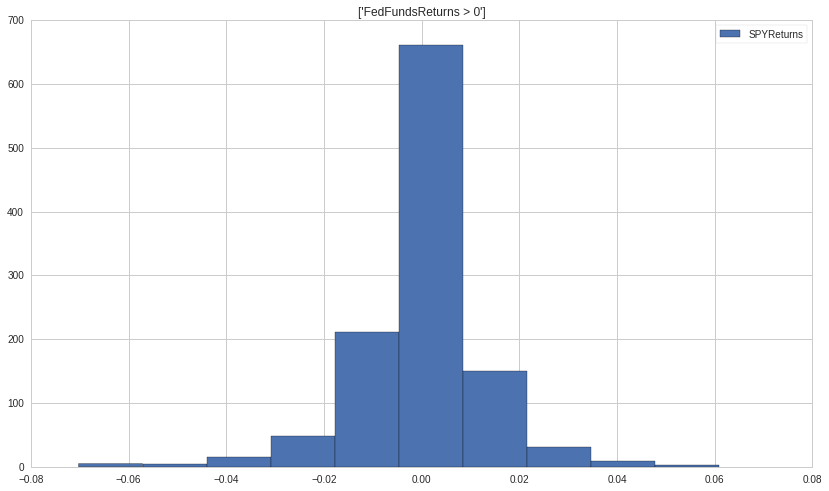There appears to be minimal skew in both distrubtions. However, they seem to be leptokurtic. Check to see if the kurtosis values are signifacntly greater than 3. Compare their SD as well.

In :
summary(v1)

Mean:   0.000333835117156
SD:     0.00900002380108
Skew:   0.555260806029
Kurt:   22.8732030624
Median: 0.0
min   -0.092260
25%   -0.000839
50%    0.000000
75%    0.002231
max    0.114975
Name: SPYReturns, dtype: float64

In :
summary(v2)

Mean:   -1.95137047118e-05
SD:     0.0128295168391
Skew:   -0.565991468247
Kurt:   4.91774804023
Median: 0.000705833631525
min   -0.070286
25%   -0.004748
50%    0.000706
75%    0.005736
max    0.060862
Name: SPYReturns, dtype: float64


Before we do our F-test, we should test our assumption of normality in the two variables. The distributions look pretty normal but let's try a jarque-bera to confirm. The F-test compares variances, and variance is a good measure of dispersion in data only if the data is truly normal. So if the data is not normal, then we should not use variance as a comparison. We will alternately use a measure more robust to skew, kurtosis, etc.

In :
def normal_test(x):
_, pvalue, _, _ = jarque_bera(x)
if pvalue > 0.05:
print 'The variable is likely normally distributed.'
else:
print 'The variable is likely not normally distributed.'
normal_test(v1)
normal_test(v2)

The variable is likely not normally distributed.
The variable is likely not normally distributed.


Since the variables are not normally distributed, we should not rely on an F-test, which may be too sensitive to the non-normality. Here is an look at to what the F-test would produce.

Our alpha-level is set at: p = 0.05

In :
alpha = 0.05
n1 = len(v1)
sd1 = v1.std()
n2 = len(v2)
sd2 = v2.std()
print "Group 1, Interest-Rate Change <= 0:"
print "n1 = " + str(n1)
print "SD1 = " + str(sd1)
print "Group 2, Interest-Rate Change > 0:"
print "n2 = " + str(n2)
print "SD2 = " + str(sd2)

Group 1, Interest-Rate Change <= 0:
n1 = 4339
SD1 = 0.00900002380108
Group 2, Interest-Rate Change > 0:
n2 = 1140
SD2 = 0.0128295168391

In :
var1 = sd1**2
var2 = sd2**2
test_stat = var1/var2
print "Test-statistic, or variance ratio, is: " + str(test_stat)

Test-statistic, or variance ratio, is: 0.492115125635


If this test-statistic falls within our rejection region (below our F-critical value), then we would reject the null hypothesis and accept the alternative that the variances were significantly different.

In :
f_crit = stats.f.ppf(1-alpha, (n1-1), (n2-1))
if test_stat > f_crit:
print "We reject the null hypothesis because our test_stat of " + str(test_stat) + \
" is greater than our f_crit of " + str(f_crit) + " at alpha " + str(alpha)
else:
print "We accept the null hypothesis because our test_stat of " + str(test_stat) + \
" is less than our f_crit " + str(f_crit) + " at alpha " + str(alpha)

We accept the null hypothesis because our test_stat of 0.492115125635 is less than our f_crit 1.08165190087 at alpha 0.05


However as we stated before, the F-test is not reliable in this case. I tried it out above just to see the results anyways. Levene's test is a popular and more robust alternative. Some information on Levene's test: https://docs.scipy.org/doc/scipy-0.14.0/reference/generated/scipy.stats.levene.html http://www.statisticshowto.com/levene-test/ http://www.people.vcu.edu/~wsstreet/courses/314_20033/Handout.Levene.pdf

Levene's test takes in three parameters. First the samples whose variances we want to compare. Second we input the center we want to use (mean, median, or trimmed) median is for heavy skew, mean is for moderate tails/moderately normal, trimmed is for heavy tails. Third we input how much trimming we potentially want.

In :
# It is important to note that with the Levene test one does not want to run large
# sample sizes (or the population) because the test_stat gets impacted heavily with
# the degrees of freedom it accounts for (the test_stat will rise greatly)
# Taking samples of 5% the v1 and v2 lengths, we run the levene test on their variances
test_stat, p_value = stats.levene(v1.sample(len(v1)/20), v2.sample(len(v2)/20), center='mean')
# N is the combined sum length of all samples
N = len(v1)*20
# K is essentially the degrees of freedom allowed for all the 'treatments' (in this case, interest rates
# going up or staying constant/decreasing)
k = 2

# Find f_crit
f_crit = stats.f.ppf(alpha,(k-1),(N-k))

In :
if test_stat > f_crit:
print "We reject the null hypothesis because our test_stat of " + str(test_stat) + \
" is greater than our f_crit of " + str(f_crit) + " at alpha " + str(alpha) + \
" with p-value " + str(p_value)
else:
print "We accept the null hypothesis because our test_stat of " + str(test_stat) + \
" is less than our f_crit " + str(f_crit) + " at alpha " + str(alpha) + \
" with p-value " + str(p_value)

We reject the null hypothesis because our test_stat of 11.6630324219 is greater than our f_crit of 0.00393216274544 at alpha 0.05 with p-value 0.000735207466433


With the Levene test, we have now rejected the null hypothesis (that the variance of our samples are equal). After running the test many times, the null hypothesis is very rarely accepted on 5% sample sizes. This indicates that the variance of the underlying population v2 (days with rising interest rates) is unequal to the variance of population v1 (days with constant or decreasing interest rates).

Conclusion: Reject Null, Accept Alternative Hypothesis

- Variance is greater for overall market returns (SPY returns) on days with rising interest rates than variance for market returns is on days with constant or decreasing interest rates

Applications:

1) This could have some informational value when looking into short-term risk parity

- Some risk parity strategies may assume a constant or mean volatility for various instruments. By understanding on a daily basis that volatility may be greater than certain adjustments should be made in portfolio allocation and expected returns

2) This conclusion could be valuable for options sellers, who collect greater premium based on greater volatility.

Source for Fed Funds Data: https://fred.stlouisfed.org/graph/?id=DFF,

In [ ]: